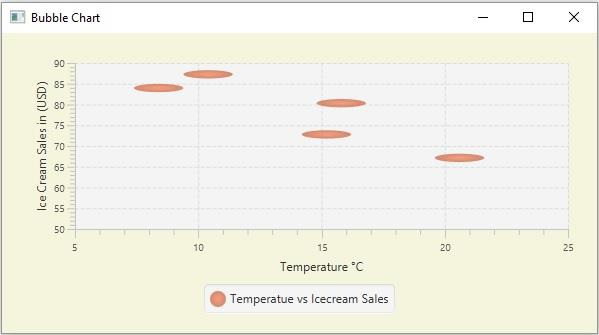# How to create a bubble chart with two parameters in JavaFX?

The bubble chart accepts a series of data points (x, y) as input values and, creates bubbles for the data points in the given series. In JavaFX, you can create a bubble chart by instantiating the javafx.scene.chart.BubbleChart class.

Generally, in all X-Y charts, the data points plot two values (x, y). In the bubble chart, there is a third value which is represented by the radius of the bubble. This chart comes handy while plotting the data points in 3 dimensions.

Anyhow, it is not mandatory to have the third value, it is only optional. Like any other XY chart, you can create a bubble chart with two values.

## Example

Following is a JavaFX example demonstrating, the creation of a bubble chart with two values −

import javafx.application.Application;
import javafx.geometry.Insets;
import javafx.scene.Scene;
import javafx.stage.Stage;
import javafx.scene.chart.BubbleChart;
import javafx.scene.chart.NumberAxis;
import javafx.scene.chart.XYChart;
import javafx.scene.layout.StackPane;
public class BubbleChart_TwoParams extends Application {
public void start(Stage stage) {
//Creating the X and Y axes
NumberAxis xAxis = new NumberAxis(5, 25, 5);
NumberAxis yAxis = new NumberAxis(50, 90, 5);
//Setting labels to the axes
xAxis.setLabel("Temperature °C");
yAxis.setLabel("Ice Cream Sales in (USD)");
//Creating the Scatter chart
BubbleChart bubbleChart = new BubbleChart(xAxis, yAxis);
//Preparing data for the scatter chart
XYChart.Series series = new XYChart.Series();
//Setting the data to scatter chart
//Setting title to the scatter chart
//scatterChart.setTitle("Ice Cream Sales vs Temperature");
//Setting name to the series
series.setName("Temperatue vs Icecream Sales");
//Creating a stack pane to hold the chart
StackPane pane = new StackPane(bubbleChart);
pane.setStyle("-fx-background-color: BEIGE");
//Setting the Scene
Scene scene = new Scene(pane, 595, 300);
stage.setTitle("Bubble Chart");
stage.setScene(scene);
stage.show();
}
public static void main(String args[]){
launch(args);
}
}

## Output